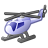Announcements
The way to achieve your own success is the willingness to help somebody else. Go for it!
cancel
Showing results for
Did you mean:Contributor III

## In Script Take result of variable and put into a field then output to QVD

I have a variable(vVAR01) which contains a calculation Example (=FieldA + FieldB). In the script I want to take the RESULT of this variable (FIELDA = 2 FIELDB = 3) Result = 5  and put it into a Field which can be output to a QVD.?  ie output the contents of result.

1 Solution

Accepted SolutionsContributor III
Author

My original variable contents was          = (C2/C1*100)     Using your logic my result was '=(C2/C1*100)'

where as I was requiring the value   97.5564

I have now found that you can use the 'EVALUATE'    function EG

Evaluate (\$(vSR283)) AS Result

Where the variable is loaded via a spreadsheet and contains the above code.  (C2/C1*100)

2 RepliesMaster III

May be like this.?

Let A=5;
Let B=10;
Let Result=A+B;
Let Result1=A*B;

New:
LOAD  '\$(Result)' as Result,'\$(Result1)' as Result1 Inline [
Temp
''
]
;

STORE New into Variable_store.qvd(qvd);Contributor III
Author

My original variable contents was          = (C2/C1*100)     Using your logic my result was '=(C2/C1*100)'

where as I was requiring the value   97.5564

I have now found that you can use the 'EVALUATE'    function EG

Evaluate (\$(vSR283)) AS Result

Where the variable is loaded via a spreadsheet and contains the above code.  (C2/C1*100)Community Browser